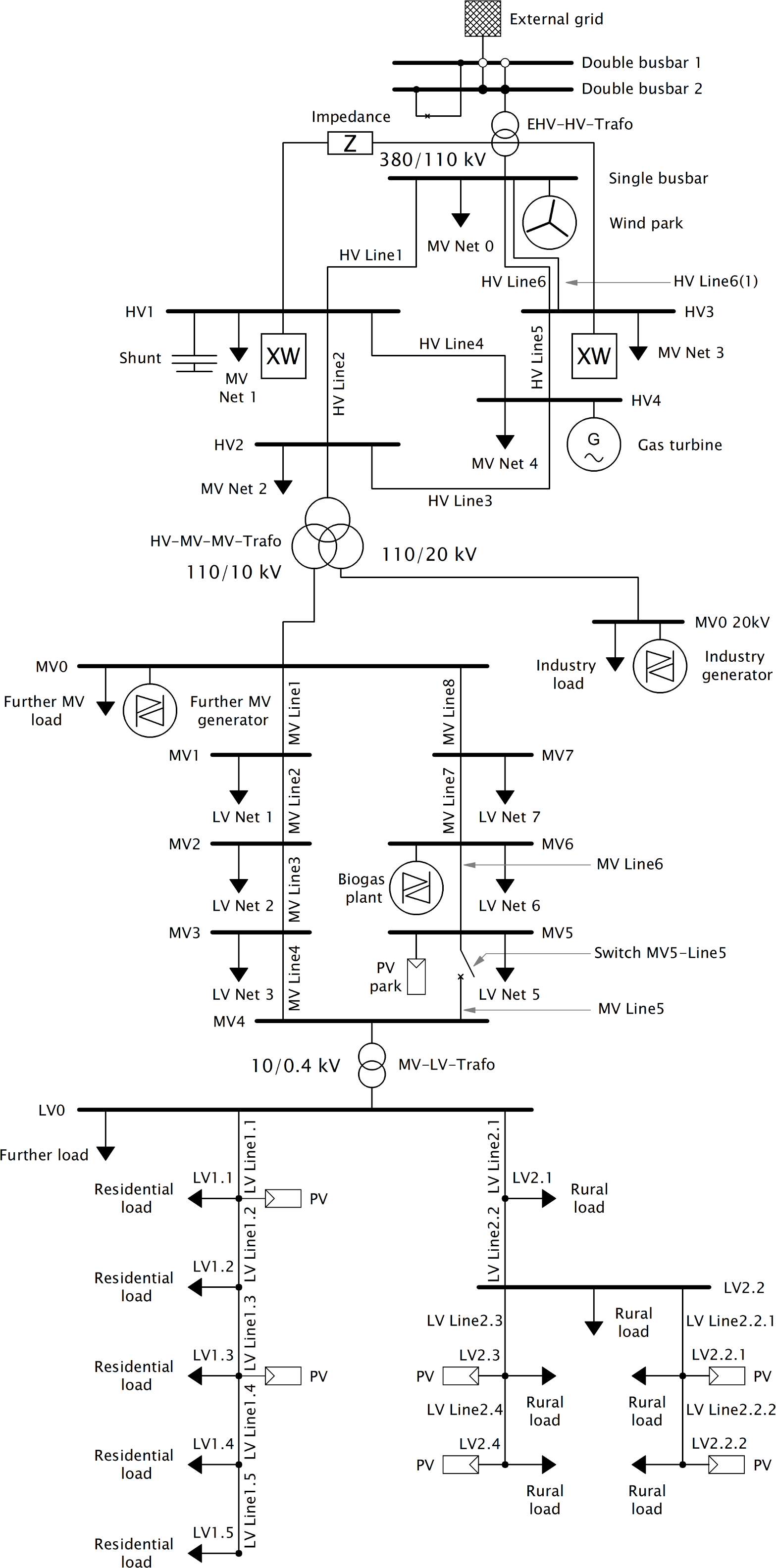# Example Networks

There are two example networks available. The simple example network shows the basic principles of how to create a pandapower network. If you like to study a more advanced and thus more complex network, please take a look at the more multi-voltage level example network.

## Simple Example Network

The following example contains all basic elements that are supported by the pandapower format. It is a simple example to show the basic principles of creating a pandapower network.

pandapower.networks.example_simple()

Returns the simple example network from the pandapower tutorials.

OUTPUT:

net - simple example network

EXAMPLE:

```>>> import pandapower.networks
>>> net = pandapower.networks.example_simple()
```The stepwise creation of this network is shown in the pandapower tutorials.

## Multi-Voltage Level Example Network

The following example contains almost all elements that are supported by the pandapower format. It is a more realistic network than the simple example and of course more complex. Using typically voltage levels for european distribution networks (high, medium and low voltage) the example relates characteristic topologies, utility types, line lengths and generator types spread over the voltage levels. To set network size limits the quantity of nodes in every voltage level is restricted and one medium voltage open ring and only two low voltage feeder are considered. Other feeders are represented by equivalent loads. As an example one double busbar and one single busbar are considered.

pandapower.networks.example_multivoltage()

Returns the multivoltage example network from the pandapower tutorials.

OUTPUT:

net - multivoltage example network

EXAMPLE:

```>>> import pandapower.networks
>>> net = pandapower.networks.example_multivoltage()
```The stepwise creation of this network is shown in the pandapower tutorials.